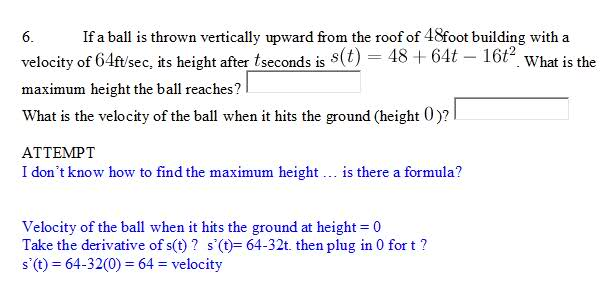# Related Rates problem

asdfsystemaI believe this is a related rates problem.

I attempted part 2 but I'm not sure about the equation for the maximum height. is it the derivative ?

## Answers and Replies

Mentor
This isn't a related rates problem. The height of the ball is given by the function s(t). The ball goes straight up, slowing as it goes, and momentarily stops. After that, it falls straight down.

The function you're given describes the height of the ball relative to time. What is the shape of this graph? The high point of the graph tells you the height of the ball at its highest position, and the time when it gets there.

For the second part, your derivative is correct, but if you evaluate the derivative at t = 0, what you're getting is the velocity at t = 0. IOW, the velocity when the ball is thrown.

Use your given function to find when s(t) = 0. Whatever time you get is the time when the ball hits the ground. Use that value in your velocity function to find the ball's velocity when it hits.

luju
Find derivative of s(t) and set it equal to zero, and then solve for time. The time you get when you do this tells you when the ball stops moving going up, in other words this is the time when your balls final velocity is zero. Take this time and plug it back to your s(t) eqution and it tells you the maximum height of the ball.

asdfsystema
part A.

it is a parabola with a max , so the maximum height will be at the max point in the graph.

s(t) = 48t+64t-16t^2
take derviative . s'(t) = 64 -32t
set to zero t= 2 . plug t back in original?

48(2)+64(2)-16(2)^2 = 160

Part B.

i did what you suggested.
s(t) = 48+64t-16t^2 = 0

I get -(t+1) (t+4) sooo t= -1 and t=-4 ??

i know what to do next but im getting two "t" values right now ..

Mentor
part A.

it is a parabola with a max , so the maximum height will be at the max point in the graph.

s(t) = 48t+64t-16t^2
take derviative . s'(t) = 64 -32t
set to zero t= 2 . plug t back in original?

48(2)+64(2)-16(2)^2 = 160
To clarify what you wrote,
s'(t) = 0 ==> t = 2
s(2) = 160
Your calculations are correct, but what are the units here? Many instructors require that you state your answer in words.
Part B.

i did what you suggested.
s(t) = 48+64t-16t^2 = 0

I get -(t+1) (t+4) sooo t= -1 and t=-4 ??
You started with an equation: 48 + 64t - 16t^2 = 0.
Each step should be an equation.
If I multiply the factors you show, I get -t^2 - 5t -4. Is that related to what you started with?
i know what to do next but im getting two "t" values right now ..

asdfsystema
Oops sorry I was posting the wrong equation haha.

I meant (-t-1)(t+3)

which will give me -t^2-4t-3 and is the same as -16t^2+64t+48 after dividing the whole thing by 16.

What is my next step ?

Mentor
Your next step is to go back and factor the left side of the equation -16t^2 +64t + 48 again. This time, try to get the right factors.
Also, each step should be an equation.

asdfsystema
ok i factored it again

x= 4.6457
x= -0.6457

What do I do next ... ?

Mentor
OK, now you have the right numbers.
What do they represent in terms of this problem? (You have them as values of x, but the original equation doesn't involve x.)

As for "what do I do next?" look at the original problem and try to understand what it is that you are doing. What you have done is find values for which s(t) = 0. Do you understand why you needed to do that, and what you have to do next? If you don't, take a look at message #2.

asdfsystema
s(t) = 48+64t-16t^2

v(t)= 64-32t

v(4.6457)= 64-32(4.6457)

v(t) = -84.6624

is that correct ?

Mentor
The numbers look about right. What are the units?